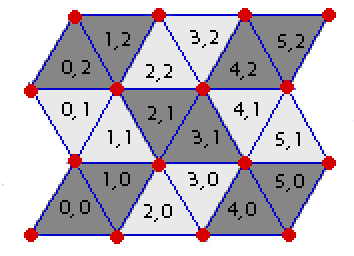시간 제한메모리 제한제출정답맞힌 사람정답 비율
2 초 512 MB222100.000%

## 문제In the game of Rhombinoes, you have a board made up entirely of equilateral trianges (see the image), some of which are "live" and some are "dead". Your goal is to place down as many rhombinoes ("rhombus"-shaped pieces) as possible on the board. Each rhombino should exactly cover two "adjacent" live triangles that have a common side, and no two rhombinoes can use the same triangle.

Given the description of the live and dead triangles of a Rhombino board, what is the maximum number of rhombinoes you can simultaneously place down on the board?

Description of Board

Each triangle in the board has a pair of coordinates (x, y). The bottom-left triangle has coordinates (0, 0) and will always be a triangle with its tip pointed upward. For any given triangle with coordinates (x, y), the triangle adjacent to it on its right-side (if any) has coordinates (x+1, y), and the triangle adjacent to it on its top-side (if any) has coordinates (x, y+1). Left-side and bottom-side adjacency are defined similarly.

Each board has a width W and a height H. A board with width W and height H is the board which consists of all triangles with coordinates (x, y) such that 0 ≤ x < W and 0 ≤ y < H. For example, the game board in the image has width 6 and height 3.

(See the image for clarification.)

## 입력

The first line of input contains three space-separated integers WH, and K.

W is the width of the board, H is the height, and K is the number of dead triangles on the board (1 ≤ W ≤ 1001 ≤ H ≤ 1001 ≤ K ≤ W*H ≤ 1000).

Exactly K lines will follow. Each such line will contain a pair of space-separated integers x and y (0 ≤ x < W, 0 ≤ y < H), indicating that the triangle with coordinates (x,y) is a dead triangle. All other triangles are live.

## 출력

Output a line containing a single integer, the maximum number of rhombinoes you can simultaneously place down on the board.

## 예제 입력 1

6 3 4
1 1
2 2
4 1
3 0


## 예제 출력 1

5


## 힌트

This is the board in the image, with cells (1, 1)(2, 2)(4, 1), and (3, 0) dead.